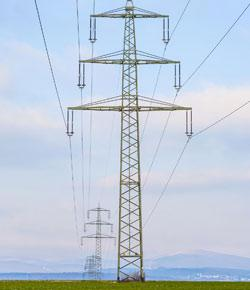# Atoms, Electric Charge, And Other Things.

14 Questions | Total Attempts: 1037SettingsA normal atom has a neutral charge with equal numbers of positive and negative particles. To find the ionic charge of an element you'll need to consult your Periodic Table metals will be positive where as Non-metals will be negative. Test what knowledge you have on charges by taking the quiz below.

• 1.
Protons are...
• A.

Negative

• B.

Neutral

• C.

Positive

• D.

Unequal

• E.

• 2.
Electrons are...
• A.

Negative

• B.

Neutral

• C.

Positive

• D.

Unequal

• E.

Weird

• 3.
The law of electric charges states that...
• 4.
What is an electric force?
• 5.
What are the two things that decide on how powerful an electric force is?
• A.

The amount of charge each object has and the amount of energy the objects have.

• B.

The amount of charge on each object and the distance between the objects.

• C.

The distance between each object and the energy each object has.

• D.

The number of objects and the number of charges.

• 6.
Do atoms have a charge?
• A.

Yes

• B.

No

• C.

Sometimes

• D.

Maybe

• E.

No idea

• 7.
What is an electric field?
• 8.
Which three of the following are ways to charge an object?
• A.

Dodkfjduction

• B.

Friction

• C.

Conduction

• D.

Sendorction

• E.

Induction

• 9.
CONSERVATION OF CHARGECharges are never....
• 10.
Why do we use an electroscope?
• A.

Because we want to know how things change over time.

• B.

Because we want to know why things are electrical.

• C.

Because we want to know if an object is charged or not.

• 11.
Can you tell if an object is negatively charged or positively charged with an electroscope?
• A.

Yes, because electroscopes behave differently.

• B.

Yes, because you can find out by checking the rim.

• C.

Sometimes, if you look closely.

• D.

No, because the electroscope is stupid.

• E.

No, because the electroscope behaves the same way

• 12.
Which of the following makes a good electrical insulator? Why?
• A.

Glass, because it is slippery and smooth.

• B.

Glass, copper, and wood, because electrons cannot move freely inside them.

• C.

Glass, wood, and plastic, because electrons cannot move freely inside them.

• D.

Copper, because it is sparky.

• 13.
What makes a good electrical conductor? Why?
• A.

Copper, glass, and aluminum, because their electrons can move freely.

• B.

Copper, mercury, and aluminum, because their electrons can move freely.

• C.

Glass, plastic, and cardboard because their electrons cannot move freely.

• 14.
What is static electricity?
• A.

Static, unmoving particles.

• B.

Moving electricity.

• C.

Electric charge at rest; generally produced by friction or induction

Related TopicsBack to top